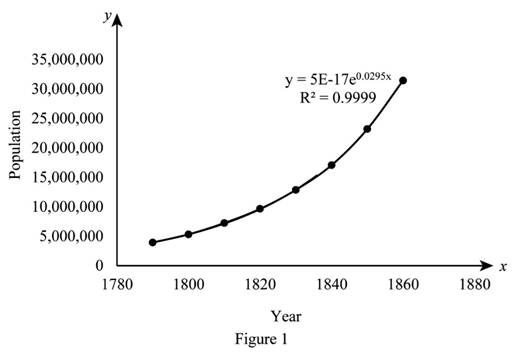# The given data points and the exponential model.### Single Variable Calculus: Concepts...

4th Edition
James Stewart
Publisher: Cengage Learning
ISBN: 9781337687805### Single Variable Calculus: Concepts...

4th Edition
James Stewart
Publisher: Cengage Learning
ISBN: 9781337687805

#### Solutions

Chapter 3.4, Problem 78E

(a)

To determine

## To sketch: The given data points and the exponential model.

Expert Solution

### Explanation of Solution

The excel calculator is used to draw the given data points and the exponential model below as shown in Figure 1.From Figure 1, it is observed that R2=0.999 that is closer to 1.

Hence, the fit is good since R2=0.999 that is closer to 1.

(b)

To determine

### To estimate: The rates of population growth in 1800 and 1850 by averaging slopes of the secant lines.

Expert Solution

The average slope of the secant lines are M1=165,550 for 1800 and M2=719,000 for 1850.

### Explanation of Solution

Formula used:

The slope of a line at two points (x0,f(x0)) and (x1,f(x1)) is, m=f(x0)f(x1)x0x1.

Calculation:

Obtain the average slope of the secant line.

For 1800, the slope between 1790 to 1800 is computed as follows,

m1=5308000392900018001790=137900

The slope between 1810 to 1800 is computed as follows,

m2=7240000530800018101800=193200

The average slope of the secant line is,

M1=m1+m22=137900+1932002=165550

Thus, the average slope of the secant line is, M1=165550.

For 1850, the slope between 1850 to 1840 is computed as follows,

m3=231920001706300018501840=612900010=612900

For 1850, the slope between 1860 to 1850 is computed as follows,

m4=314430002319200018601850=825100010=825100

The average slope of the secant line is,

M2=m3+m42=612900+8251002=14380002=719000

Thus, the average slope of the secant line is M2=719,000.

Therefore the average slope of the secant lines are M1=165,550 for 1800 and M2=719,000 for 1850.

(c)

To determine

### To estimate: The rate of growth in 1800 and 1850 by using part (a) and compare in part (b).

Expert Solution

The rate of growth in 1800 and 1850 are f(x)=169757 and f(x)=742016.

### Explanation of Solution

The derivative of the fit equation is f(x)=(1.475×1018)(1.02994)x.

Substitute x=1800 in the above equation,

f(1800)=(1.475×1018)(1.02994)1800=1.4751.0299418001018=1.475(115203.445)=169925

Substitute x=1850 in f(x).

f(1850)=(1.475×1018)(1.02994)1850=1.4751.0299418501018=1.475(503572.18319)742769

Therefore, the rate of growth in 1800 and 1850 are f(x)=169,925 and f(x)=742,769.

(d)

To determine

Expert Solution

### Explanation of Solution

The value of the exponential model to predict the population in 1870 is given by 45,375,883 but the actual value is 38,558,000.

Thus, value of the exponential model to predict the population in 1870 is much larger than the actual value 38,558,000 because the model used is simplistic.

This model is basically assigns population to dates at 10 year intervals and used to predict a rough answer, but cannot predict with uncertainty

### Have a homework question?

Subscribe to bartleby learn! Ask subject matter experts 30 homework questions each month. Plus, you’ll have access to millions of step-by-step textbook answers!